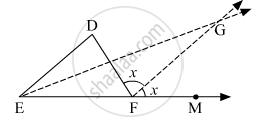Advertisement Remove all ads

# In the Given Figure, Line De || Line Gf Ray Eg and Ray Fg Are Bisectors of ∠ Def and ∠ Dfm Respectively. Prove That, - Geometry

Sum

In the given figure, line DE ||   line GF ray EG and ray FG are bisectors of ∠ DEF and ∠ DFM respectively. Prove that,

1. angle DEG = 1/2  angle EDF

2. EF = FGAdvertisement Remove all ads

#### Solution

(i) Given: DE || GF
Now, ∠ DEF = ∠ GFM              (Corresponding angles as DM is a transversal line)
⇒ 2 ∠ DEG = ∠ DFG         (Ray EG and ray FG are bisectors of ∠ DEF and ∠ DFM)
⇒ 2 ∠ DEG = ∠ EDF          (∵ ∠ EDF = ∠ DFG, alternate angles as DF is a transversal line)
⇒ ∠ DEG =1/2 EDF

(ii) Given: DE || GF
∠ DEG = ∠ EGF              (Alternate angles as EG is a transversal line)
∴ ∠ GEF = ∠ EGF            (∵ ∠ DEG = ∠ GEF)
∴ EF = FG                         (Sides opposite to equal angles)

Is there an error in this question or solution?
Advertisement Remove all ads

#### APPEARS IN

Balbharati Mathematics 2 Geometry 9th Standard Maharashtra State Board
Chapter 3 Triangles
Practice Set 3.1 | Q 10 | Page 28
Advertisement Remove all ads
Advertisement Remove all ads
Share
Notifications

View all notifications

Forgot password?